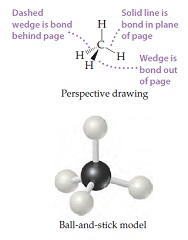# Problem: The methane molecule, CH4, has the geometry shown in following figure. Imagine a hypothetical process in which the methane molecule is "expanded," by simultaneously extending all four C–H bonds to infinity. We then have the process CH4(g) → C(g) + 4 H(g)Suppose that 3.70 g CH4(g) is reacted with 1.13 g F2(g), forming CF4(g) and HF(g) as sole products. What is the limiting reagent in this reaction?

###### FREE Expert Solution

We are asked what is the limiting reagent in this reaction.

Balanced reaction:

CH4(g) +  4 F2(g) → CF4(g) + 4 HF(g)

Calculate product using both reactants:

89% (398 ratings)###### Problem Details
The methane molecule, CH4, has the geometry shown in following figure. Imagine a hypothetical process in which the methane molecule is "expanded," by simultaneously extending all four C–H bonds to infinity. We then have the process CH4(g) → C(g) + 4 H(g)Suppose that 3.70 g CH4(g) is reacted with 1.13 g F2(g), forming CF4(g) and HF(g) as sole products. What is the limiting reagent in this reaction?

Frequently Asked Questions

What scientific concept do you need to know in order to solve this problem?

Our tutors have indicated that to solve this problem you will need to apply the Limiting Reagent concept. You can view video lessons to learn Limiting Reagent. Or if you need more Limiting Reagent practice, you can also practice Limiting Reagent practice problems.